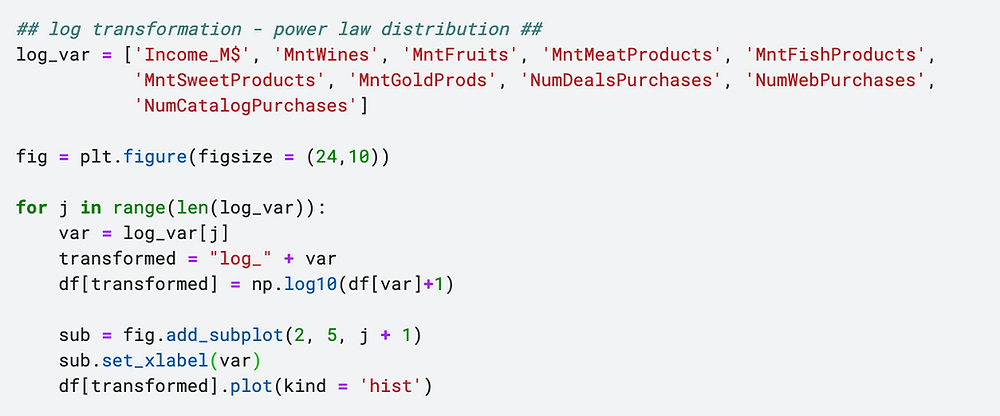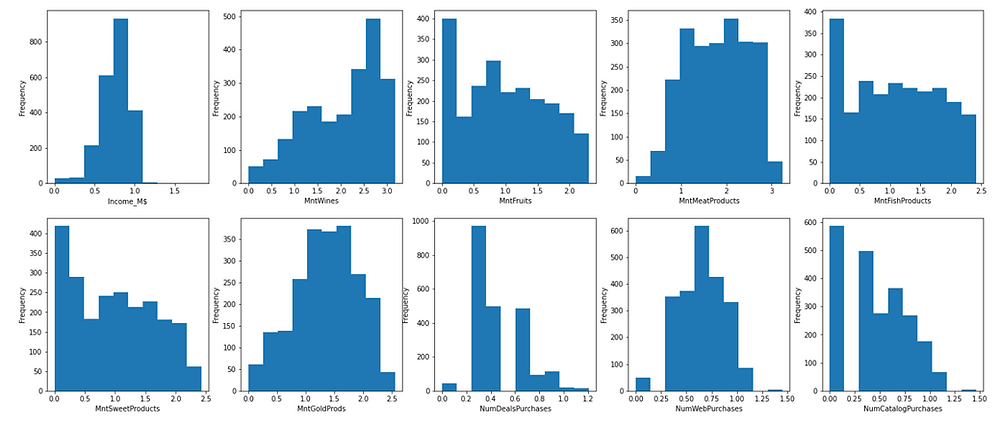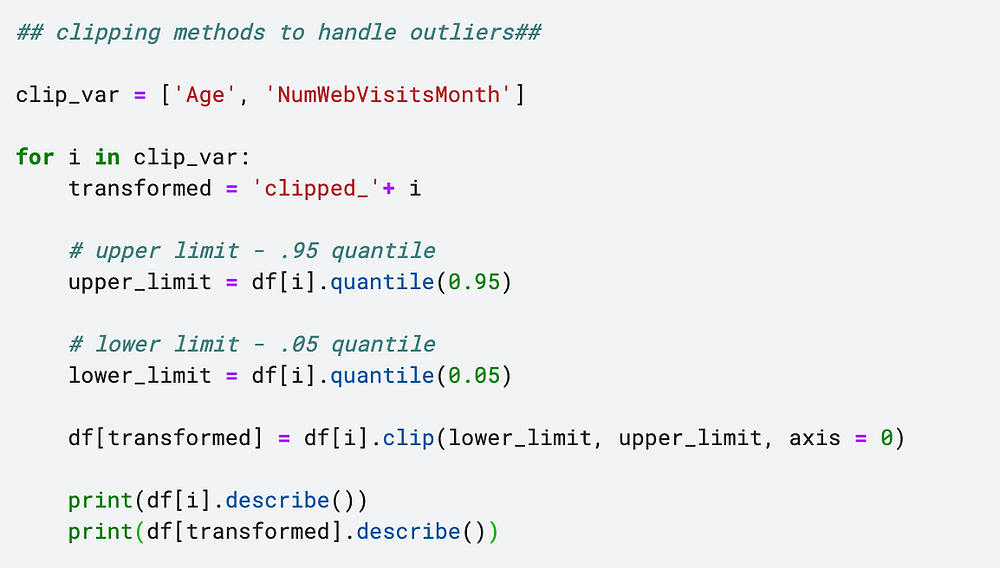Search

# Data Transformation and Feature Engineering in Python

## How to Choose the Appropriate Technique Based on Your DataData transformation is the process of converting raw data into a a format or structure that would be more suitable for the model or algorithm and also data discovery in general. It is an essential step in the feature engineering that facilitates discovering insights. This article mainly covers techniques of numeric data transformation.

Why need data transformation?

• the algorithm is more likely to be biased when the data distribution is skewed

• transforming data into the same scale allows the algorithm to compare the relative relationship between data points better

When to apply data transformation?

When implementing supervised algorithms, training data and testing data need to be transformed in the same way. This is usually achieved by feeding the training dataset to building the data transformation algorithm and then apply that algorithm to the test set.

## Feature Engineering and EDA

For this exercise, I am using the Marketing Analytics dataset from Kaggle. Firstly I performed some basic feature engineering to make data tidier and more insightful.1. transform year of birth to "Age" This is a basic subtraction of year of birth to the current year.

2. transform the date customer enrolled ("Dt_Customer") into "Enrollment_Length" This process is similar to the one above with additionally extracting the year part from the date feature.

3. transform currency ("Income") into numbers ("Income_M\$") This involves four steps: 1) clean data to remove characters ", \$ ." 2) substitute null value to 0; 3) convert string into integer; 4) scale down the numbers into million dollar which helps with visualizing the data distribution

Now, let's visualize current data distribution using a simple univariate EDA technique - histogram. It is not hard to see that most variables are heavily skewed.If you want to know more about data visualization and exploratory data analysis (EDA), please check out: Semi-Automated Exploratory Data Analysis (EDA) in Python.

## Log Transformation - right skewed data

When the data sample follows the power law distribution, we can use log scaling to transform the right skewed distribution into normal distribution. To achieve this, simply use the np.log() function. In this dataset, most variables fall under this category.After the log transformation, these features have become more normally distributed.## Clipping - handle outliers

This approach is more suitable when there are outliers in the dataset. Clipping method sets up the upper and lower bound and all data points will be contained within the range.

We can use quantile() to find out what is the range of the majority amount of data (between 0.05 percentile and 0.95 percentile). Any numbers below the lower bound (defined by 0.05 percentile) will be rounded up to the lower bound. Similarly, the numbers above upper bound (defined by 0.95 percentile) will be rounded down to upper bound.From the histogram in the EDA process, we can see that variable "Age" and "NumWebVisitsMonth" have outliers with extraordinary large numbers. So I will only apply clipping to these two columns.

Consequently, the maximum value for both fields dropped significantly:

• Age: from 128 to 71

• NumWebVisitMonth: from 20 to 8